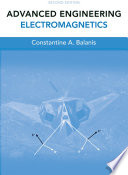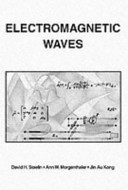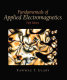### Application of Electromagnetic Waves in Engineering

Data is displayed for academic year: 2023./2024.

#### Course Description

The difference between lumped and distributed parameters, the equivalent circuit of a differential section of a transmission line, physical explanation of all the elements of the equivalent circuit. Physical explanation of the phenomenon of reflection, the equation of the current and voltage at the differential section of a transmission line. Wave equation for lossless transmission line, general form of the solution and its physical interpretation. The form of the wave equation solution for an excitation signal with sinusoidal waveform, physical interpretation. Physical interpretation of the phase velocity, group velocity and the dispersion phenomenon. The phenomenon of standing wave at the transmission line and its physical explanation. Input impedance of the losses and lossy transmission line terminated with a reactive load. Explanation of the graph that shows an impedance as a function of the location along the transmission line. Definition of a reflection coefficient, standing wave ratio, Smith chart, the principle of matching. Physical interpretation of the divergence and curl of a vector field, the basic idea of electromagnetic field. Continuity equation, displacement current, Maxwell's equations and their physical interpretation. Physical interpretation of permittivity and permeability, isotropic and anisotropic materials. Boundary conditions at the interface between two dielectrics, at the interface between the dielectric and conductor, perfect electric conductor (PEC), perfect magnetic conductor (PMC). Vector wave equation, interpretation of the solutions, the concept of plane wave. The flow of electromagnetic energy, Poynting vector, wave vector. Intrinsic impedance and a concept of the impedance. Reflection and transmission of normally incident plane waves (the media with and without losses, penetration depth). Reflection and transmission of plane waves with oblique incidence (lossless medium, TE and TM polarization). Parallel-plate waveguide, the physical interpretation of wave propagation. Rectangular waveguide, modes of propagation, wave impedance, propagation factor, phase and group velocities. Electromagnetic resonators, quality factor, degenerate modes, single-mode resonator, multi-mode resonators. Electromagnetic wave propagation in the dielectric slab. Comparison of structures for guiding of electromagnetic waves: two-wire line, coaxial line, metal waveguide, the dielectric slab, fiber, planar structures. Vector potential, the concept radiation, Hertz dipole. Basic antenna parameters, the antenna array with two radiating elements.

#### Study Programmes

[FER3-EN] Computing - study
Elective Courses (5. semester)
[FER3-EN] Electrical Engineering and Information Technology - study
Elective Courses (5. semester)

#### Learning Outcomes

1. Explain the background physics of EM propagation in free space, unbounded lossless and lossy dielectric, and in guiding structures
2. Explain physical meaning of Maxwell equations in differential and integral form, vector wave equation and its solutions for traveling wave, standing wave, and evanescent wave
3. Explain physical background of EM wave radiation of elemental electric dipole and a simple two-element antenna array
4. Compute parameters (characteristic impedance and propagation constant) of TEM transmission line, rectangular waveguide, and dielectric waveguide
5. Compute all the parameters needed for one-stub matching of general load
6. Compute field distribution in the case of normal and oblique incidence of the EM wave to general half-space
7. Identify devices for radiation and guiding of EM energy in communication and electronic engineering systems and explain background physics

#### Forms of Teaching

Lectures

All lectures contain in-front-of-class experiment that are presented before the theoretical analysis

Exercises

Four cycles of two hours

Independent assignments

Six problems assigned before each lab exercise

Laboratory

Lab exercises: three groups of 3 hours per week in departmental lab

Continuous Assessment Exam
Laboratory Exercises 100 % 10 % 100 % 10 %
Class participation 10 % 10 % 10 % 10 %
Mid Term Exam: Written 50 % 25 % 25 %
Final Exam: Written 50 % 25 %
Final Exam: Oral 50 %
Exam: Written 50 % 50 %
Exam: Oral 50 %

#### Week by Week Schedule

1. Difference between lumped elements and distributed-parameter networks, Lumped element model for a transmission line
2. Telegrapher equations; Wave equations; General solution and physical interpretation, Voltage and current waves on the transmission line; Reflection coefficient; Standing wave ratio
3. Input impedance of the lossless and lossy transmission line; Impedance along the transmission line, Characteristic impedance and propagation coefficient
4. Phase and group velocity, Power flow on the transmission line, Lossless line; Low-loss line
5. Smith chart, Single-stub tuning, Matching for maximum power transfer
6. Time domain response of the transmission line; Pulse propagation; Dispersion and causality, Transmission line with periodic loading; Artificial transmission lines
7. Physical interpretation of curl and divergence; The concept of electromagnetic field, Continuity equation; Displacement current; Maxwell equations and their physical interpretation
8. Midterm exam, Permittivity an permeability; Physical interpretation; The concepts of isotropic and anisotropic materials, Boundary conditions at the interface ; The concepts of perfect electric conductor (PEC) and perfect magnetic conductor (PMC)
9. Vector wave equation; Construction and interpretation of the solution; Plane waves in lossless and lossy unbounded media, The concepts of impedance and intrinsic impedance
10. Normal and oblique incidence of plane waves on lossless and lossy half space, TEM, TE and TM waves
11. Normal incidence of plane wave on lossless and lossy half space; Penetration depth
12. Oblique incidence of plane wave on lossless half space, TE and TM polarizations
13. Parallel plate waveguide, Rectangular waveguide
14. Circular waveguide, Dielectric waveguide
15. Final exam, Elementary radiation sources

#### Literature

Zlatko Smrkić (1990.), Mikrovalna elektronika, Školska knjigaConstantine A. Balanis (2012.), Advanced Engineering Electromagnetics, John Wiley & SonsDavid H. Staelin, Ann W. Morgenthaler, Jin Au Kong (1994.), Electromagnetic Waves, PearsonFawwaz Tayssir Ulaby (2007.), Fundamentals of Applied Electromagnetics, Prentice Hall
Juraj Bartolić (2021.), Mikrovalna elektronika, Kiklos

#### General

ID 223379
Winter semester
5 ECTS
L3 English Level
L2 e-Learning
45 Lectures
0 Seminar
15 Exercises
12 Laboratory exercises
0 Project laboratory